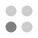• 3,149 trophies earned
• 150 Players Tracked
• 21 Total trophies
• 1,170 Points
• 147 Platinum Club
• 147 100% Club

### Energy Cycle Edge trophies

•Good job! Completed all tasks!98.67%
•Solved first puzzle100.00%
•Puzzles 2, 3 and 4 solved100.00%
•Puzzles 5, 6 and 7 solved100.00%
•Puzzles 8, 9 and 10 solved100.00%
•Puzzles 11, 12 and 13 solved100.00%
•Puzzles 14, 15 and 16 solved100.00%
•Puzzles 17, 18 and 19 solved100.00%
•Puzzles 20, 21 and 22 solved100.00%
•Puzzles 23, 24 and 25 solved100.00%
•Puzzles 26, 27 and 28 solved99.33%
•Puzzles 29, 30 and 31 solved99.33%
•Puzzles 32, 33 and 34 solved99.33%
•Puzzles 35 and 36 solved99.33%
•Puzzles 37, 38 and 39 solved99.33%
•Puzzles 40 and 41 solved99.33%
•Puzzles 42 and 43 solved99.33%
•All of puzzles solved99.33%
•Took less than 10 seconds to solve the puzzle100.00%
•Took more than 15 minutes to solve the puzzle99.33%
•3D puzzle is solved exactly with one rotation100.00%